# Oberseminar Analysis Summer 2016

## Organizers: C. Seis, S. Conti, H. Koch, S. Müller, B. Niethammer, M. Rumpf, C. Thiele, J.J.L. Velázquez

• Thursday, July 14, 3:45 p.m., R 2.040
Daoyin He (U Göttingen)
Critical exponents for semilinear Tricomi-type equations
My primary research interest lies in the semilinear generalized Tricomi equation \begin{equation*} \partial_t^2 u-t^m \Delta_x u=|u|^p \end{equation*} in $\mathbb{R}_+\times\mathbb{R}^n$ with suitable initial data $(u(0,\cdot), \partial_t u(0,\cdot))=(u_0, u_1)$, where $n\geq3$ and $m\in\mathbb{N}$. The main objective of this talk is to determine the critical exponent $p_c=p_c(m,n)$, such that if $p> p_c$, the global existence of small initial data solution is guaranteed, while, for $1 < p\leq p_c$ and (non-zero)non-negative initial data, the local solution blows up in finite time.
This problem, for the wave equation when $m=0$, was known as Strauss conjecture and was solved during the last three decades. From a physicist's point of view, the Tricomi problem is closely related to the study of gas flows with nearly sonic speed. More specifically, the Tricomi equation describes the transition from the subsonic flow (in the elliptic region) to the supersonic flow (in the hyperbolic region).
So far, in the joint work with Ingo Witt and Huicheng Yin, we have found out the precise value of the critical exponent $p_c$. As it turns out, the Tricomi operator behaves much like the wave operator. Therefore, non-weighted or weighted Strichartz estimates can establish what ultimately leads to global existence. For the blowup part, one uses a suitable formula for a modified Bessel function together with the test function method to get the result.

• Thursday, June 9, 2:15 p.m., Lipschitz-Saal
Xian Liao (U Bonn)
Global regularities of the two dimensional density patch for the inhomogeneous incompressible viscous flow
In this talk I will show the persistence of the boundary regularities of the density patch problem for the inhomogeneous incompressible Navier-Stokes equations in space dimension two, under the small jump condition. The talk will be divided into two parts. In the introduction part I will first formulate the density patch problem mathematically and then give the goal estimates. In the second part I will sketch the proof. The striated distribution spaces and the maximal regularity estimates are key tools in the proof. This is a joint work with Ping Zhang in AMSS, Beijing.

• Thursday, June 2, 2:15 p.m., Lipschitz-Saal
Matthias Erbar (U Bonn)
A gradient flow approach to the Boltzmann equation
In this talk, I will present a novel interpretation of the homogeneous Boltzmann equation (with constant collision kernel) as the gradient flow of the entropy with respect to a suitable geometry on the space of probability measures. This geometry is given by a new notion of distance between probability measures, which takes the collision process between particles into account. As a first application, one obtains a novel time-discrete approximation scheme for the homogeneous Boltzmann equation.

• Thursday, April 28, 2:15 p.m., R 2.040
Richard Kraaij (TU Delft)
Convexity of entropy along entropic interpolations
To many McKean-Vlasov equations, one can associate via stochastic approximation and large deviation theory a higher dimensional Hamiltonian dynamics. This point of view has been exploited by Mielke, Peletier, Renger  to show that if the approximating stochastic systems are reversible, then the McKean-Vlasov dynamics are a gradient flow of the limiting entropy of the stationary measures of the stochastic systems.
In this talk, we extend this point of view for (possibly non-reversible) finite dimensional systems (i.e. jump processes on finite state space, or small random perturbations of dynamical systems on subsets of R^d). We define entropic interpolations in terms of the Hamiltonian dynamics and give conditions under which the limiting entropy of the stochastic systems is convex along the entropic interpolation. We also introduce a weaker 'entropy-information' inequality that implies exponential decay of entropy along the solution of the McKean-Vlasov equation, naturally extending the notion of the modified Logarithmic Sobolev inequality for the Kolmogorov forward equation.
If time permits, we consider some simple models for which the conditions and inequalities can be verified.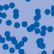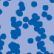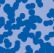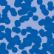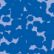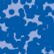## News

Florian Schweiger erhielt den Hausdorff-Gedächtnispreis 2021 der Fachgruppe Mathematik für die beste Dissertation. Er fertigte die Dissertation unter der Betreuung von Prof. Stefan Müller an. Unter anderen wurde Vanessa Ryborz mit einem Preis der Bonner Mathematischen Gesellschaft für ihre von Prof. Sergio Conti betreute Bachelorarbeit ausgezeichnet. (18.01.2022)

Prof. Dr. Sergio Albeverio has been elected into the Academia Europaea and the Accademia Nazionale dei Lincei (more; 02.12.2021).

Der SFB 1060 Die Mathematik der emergenten Effekte hat eine dritte Förderperiode erhalten. (26.11.20)

Prof. Dr. Andreas Eberle erhält den diesjährigen Lehrpreis der Universität Bonn. (22.07.2020)

Herr Dr. Richard Höfer erhielt den Hausdorff-Gedächtnispreis 2019 der Fachgruppe Mathematik für die beste Disseration. Betreut wurde die Arbeit von Prof. J. Velázquez (29.01.2020).

Contact

Managing Director: Prof. Dr. Massimiliano Gubinelli